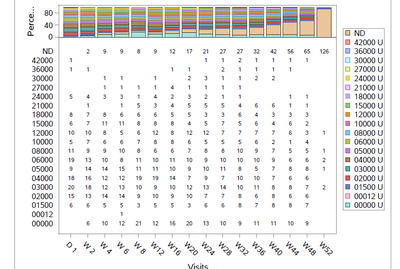## how to label the count at each stack of a stacked bar chart?

Hi

Im using the below code to create a stacked bar chart. I'm using axistable to present the n (count) for each stack , since  there a is big list of the groups ( stack) , the plot is looking compressed and not at all readable , that is, the axistable occupies most of the graphic area. What is the best way to present the count of each stack and how to do that?Any suggestion?

proc template;

define statgraph bar100;

begingraph;
layout overlay;
BARCHARTPARM X = avis y=percent / group=avalx name="lgnd1";

discretelegend "lgnd1" /halign=right across=1 sortorder=DESCENDINGFORMATTED;
innermargin / align=bottom opaque=true separator=True;
axistable x=avis value=count/ display=(label) classdisplay=stack class=avalc classorder=descending/*colorgroup=trt01p*/ position=0 pad=10 /*headerlabel='Number of subjects'*/;
endinnermargin;

endlayout;

endgraph;
end;
run;
proc sgrender data=g100 template=bar100;
run;

My output below:DanH_sas
SAS Super FREQ

## Re: how to label the count at each stack of a stacked bar chart?

Normally, you could use the SEGEMENTLABELTYPE to turn on the bar segment labels, but this will show the response values, which are the values in your "percent" variable. To show your "count" variable in the same location, your best option is to overlay a TEXTPLOT on the BARCHARTPARM to put the count values in the segments.

To do this, you will need to write a little data step to calculate the y positions for each value in a separate column that you will use for the Y column on the TEXTPLOT statement. The data step should accumulate the y positions for group value in a category, so be sure your data is sorted correctly before feed it into this data step.

Hope this helps!

Dan

Discussion stats